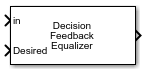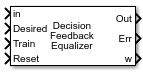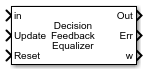# Decision Feedback Equalizer

Equalize modulated signals using decision feedback filtering

Since R2019a

•Libraries:
Communications Toolbox / Equalizers

## Description

The Decision Feedback Equalizer block uses a decision feedback filter tap delay line with a weighted sum to equalize modulated signals transmitted through a dispersive channel. Using an estimate of the channel modeled as a finite input response (FIR) filter, the block processes input frames and outputs the estimated signal.

This icon shows the block with all ports enabled for configurations that use the LMS or RLS adaptive algorithm.This icon shows the block with all ports enabled for configurations that use the CMA adaptive algorithm.## Ports

### Input

expand all

Input signal, specified as a column vector. The vector length of in must be equal to an integer multiple of the Number of input samples per symbol parameter. For more information, see Symbol Tap Spacing.

Data Types: `double`
Complex Number Support: Yes

Training symbols, specified as a column vector. The vector length of Desired must be less than or equal to the length of input in. The Desired input port is ignored when the Train input port is `0`.

#### Dependencies

To enable this port, set the Adaptive algorithm parameter to `LMS` or `RLS`.

Data Types: `double`
Complex Number Support: Yes

Train equalizer flag, specified as `1` or `0`. The block starts training when this value changes from `0` to `1` (at the rising edge). The block trains until all symbols in the Desired input port are processed.

#### Dependencies

To enable this port, set the Adaptive algorithm parameter to `LMS` or `RLS` and select the Enable training control input parameter.

Data Types: `Boolean`

Update tap weights flag, specified as `1` or `0`. The tap weights are updated when this value is `1`.

#### Dependencies

To enable this port, set the Adaptive algorithm parameter to `CMA` and the Source of adapt weights flag parameter to `Input port`.

Data Types: `Boolean`

Reset equalizer flag, specified as `1` or `0`. If Reset is set to `1`, the block resets the tap weights before processing the incoming signal. The block performs initial training until all symbols in the Desired input port are processed.

#### Dependencies

To enable this port, select the Enable reset input parameter.

Data Types: `Boolean`

### Output

expand all

Equalized symbols, returned as a column vector that has the same length as input signal in.

This port is unnamed until you select the Output error signal or Output taps weights parameter.

Error signal, returned as a column vector that has the same length as input signal in.

Tap weights, returned as an NTaps-by-1 vector, where NTaps is equal to the sum of the Number of forward taps and Number of feedback taps parameter values. w contains the tap weights from the last tap weight update.

## Parameters

expand all

Structure parameters

Number of forward equalizer taps, specified as a positive integer. The number of forward equalizer taps must be greater than or equal to the value of the Number of input samples per symbol parameter.

Number of feedback equalizer taps, specified as a positive integer.

Signal constellation, specified as a vector. The default value is a QPSK constellation generated using this code: `pskmod(0:3,4,pi/4)`.

Number of input samples per symbol, specified as a positive integer. Setting this parameter to any number greater than `1` effectively creates a fractionally spaced equalizer. For more information, see Symbol Tap Spacing.

Algorithm parameters

Adaptive algorithm used for equalization, specified as one of these values:

Step size used by the adaptive algorithm, specified as a positive scalar. Increasing the step size reduces the equalizer convergence time but causes the equalizer output estimates to be less stable.

Tunable: Yes

#### Dependencies

To enable this parameter, set Adaptive algorithm to `LMS` or `CMA`.

Forgetting factor used by the adaptive algorithm, specified as a scalar in the range (0, 1]. Decreasing the forgetting factor reduces the equalizer convergence time but causes the equalizer output estimates to be less stable.

Tunable: Yes

#### Dependencies

To enable this parameter, set Adaptive algorithm to `RLS`.

Initial inverse correlation matrix, specified as a scalar or an NTaps-by-NTaps matrix. NTaps is equal to the sum of the Number of forward taps and Number of feedback taps parameter values. If you specify this value as a scalar, a, the equalizer sets the initial inverse correlation matrix to a times the identity matrix: a(`eye`(NTaps)).

#### Dependencies

To enable this parameter, set Adaptive algorithm to `RLS`.

Control parameters

Reference tap, specified as a positive integer less than or equal to the Number of forward taps parameter value. The equalizer uses the reference tap location to track the main energy of the channel.

Input signal delay in samples relative to the reset time of the equalizer, specified as a nonnegative integer. If the input signal is a vector of length greater than 1, then the input delay is relative to the start of the input vector. If the input signal is a scalar, then the input delay is relative to the first call of the block and to the first call of the block after the Reset input port toggles to `1`.

#### Dependencies

To enable this parameter, set Adaptive algorithm to `LMS` or `RLS`.

Source of the adapt tap weights request, specified as one of these values:

• `Property` — Specify this value to use the Adaptive algorithm parameter to control when the block adapts tap weights.

• `Input port` — Specify this value to use the Update input port to control when the block adapts tap weights.

#### Dependencies

To enable this parameter, set Adaptive algorithm to `CMA`.

Select this parameter to adaptively update the equalizer tap weights. If this parameter is cleared, the block keeps the equalizer tap weights unchanged.

#### Dependencies

To enable this parameter, set Adaptive algorithm to `CMA` and Source of adapt weights flag to `Property`.

Source for initial tap weights, specified as one of these values:

• `Auto` — Initialize the tap weights to the algorithm-specific default values, as described in the Initial weights parameter.

• `Property` — Initialize the tap weights using the Initial weights parameter value.

Initial tap weights used by the adaptive algorithm, specified as a scalar or an NTaps-by-1 vector. NTaps is equal to the sum of the Number of forward taps and Number of feedback taps parameter values. The default is `0` when the Adaptive algorithm parameter is set to `LMS` or `RLS`. The default is `[0;0;1;0;0]` when the Adaptive algorithm parameter is set to `CMA`.

If you specify Initial weights as a vector, the vector length must be NTaps. If you specify Initial weights as a scalar, the equalizer uses scalar expansion to create a vector of length NTaps with all values set to Initial weights.

#### Dependencies

To enable this parameter, set Initial tap weights source to `Property`.

Tap weight update period in symbols, specified as a positive integer. The equalizer updates the tap weights after processing this number of symbols.

Select this parameter to enable input port Train. If this parameter is cleared, the block does not reenter training mode after the initial tap training.

#### Dependencies

To enable this parameter, set Adaptive algorithm to `LMS` or `RLS`.

Select this parameter to use decision directed mode to update equalizer tap weights. If this parameter is cleared, the block keeps the equalizer tap weights unchanged after training.

#### Dependencies

To enable this parameter, set Adaptive algorithm to `LMS` or `RLS`.

Select this parameter to enable input port Train. If this parameter is cleared, the block does not reenter training mode after the initial tap training.

Diagnostic parameters

Select this parameter to enable output port Err containing the equalizer error signal.

Select this parameter to enable output port w containing tap weights from the last tap weight update.

Type of simulation to run, specified as `Code generation` or `Interpreted execution`.

• `Code generation` — Simulate the model by using generated C code. The first time you run a simulation, Simulink® generates C code for the block. The model reuses the C code for subsequent simulations unless the model changes. This option requires additional startup time, but the speed of the subsequent simulations is faster than with the `Interpreted execution` option.

• `Interpreted execution` — Simulate the model by using the MATLAB® interpreter. This option shortens startup time, but the speed of subsequent simulations is slower than with the ```Code generation``` option. In this mode, you can debug the source code of the block.

## Block Characteristics

 Data Types `double` | `single` Multidimensional Signals `no` Variable-Size Signals `yes`

expand all

expand all

## Version History

Introduced in R2019a